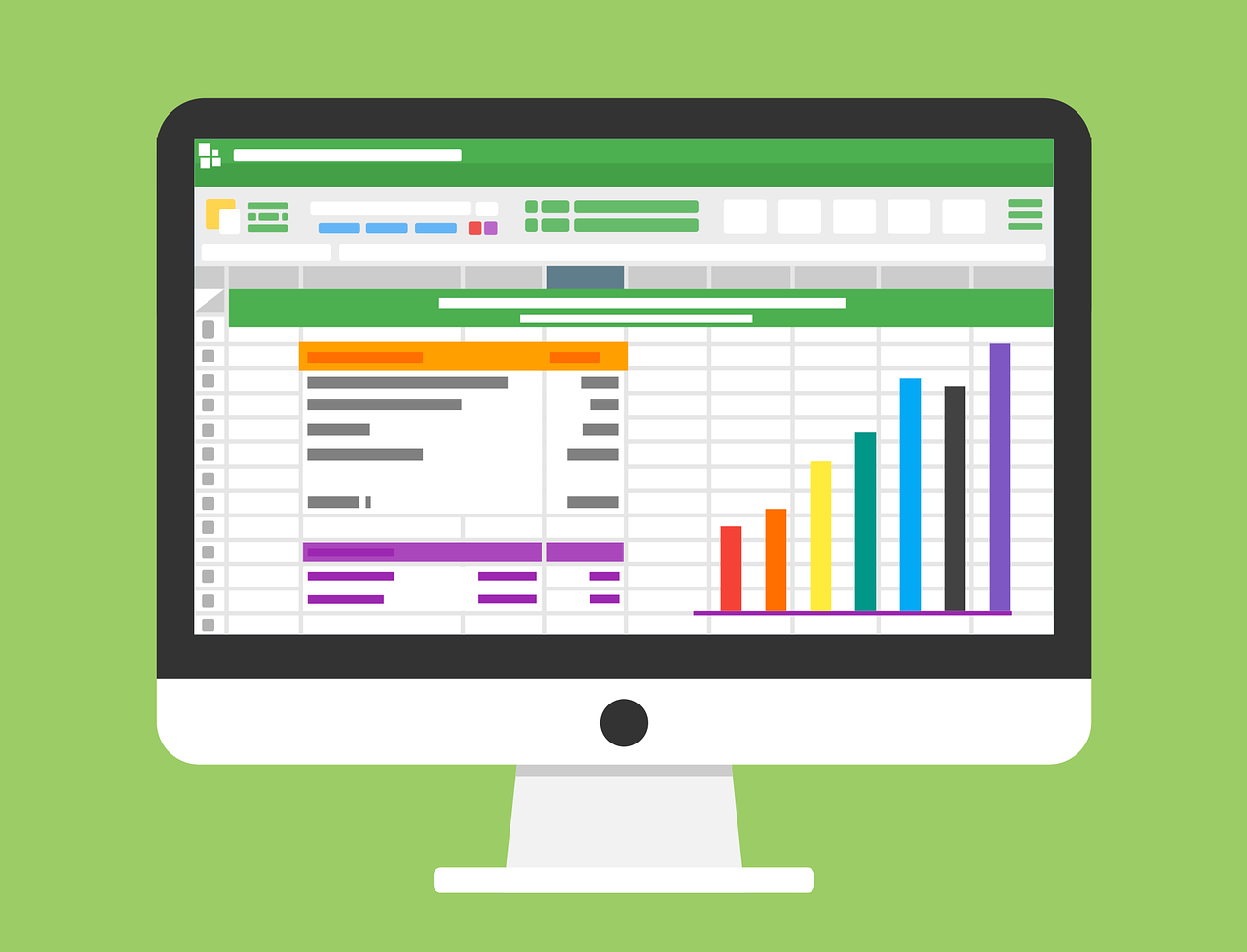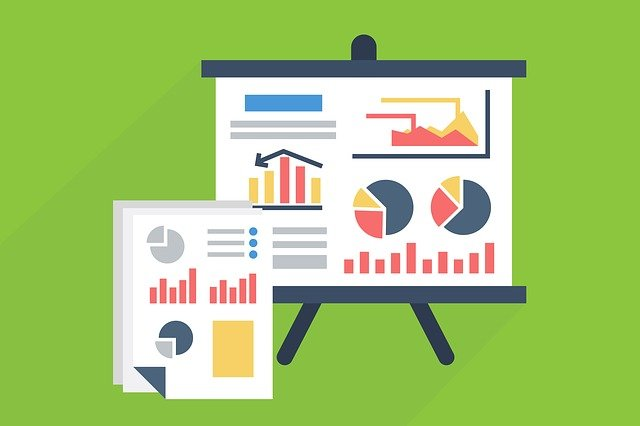Math Concepts
Breaking down the myth of "Is Statistics hard?"
26.6k views

 1 Introduction 2 Is Statistics Hard 3 Why is statistics so hard? 4 Summary 5 FAQs 6 External references

29 October  2020

## Introduction

Is statistics hard? How hard is statistics?

Is statistics easy?

How to get better at statistics?

We encounter this question a lot from students. The answer is not so straight forward. Let us start by defining what statistics is.

Statistics is the science of collecting, organizing, analyzing, and interpreting data. Yes, it is all about data!In the 21st century, data is a vital asset. Companies pour millions of dollars to collect and analyze their customer data. Data is our footprint on the internet. .

Due to the importance of data in today's world, statistics is taught right from the schooldays. A good base in statistics can open a wide variety of career doors for a student.

Many times, statistics become a bit difficult to handle for students, and they may be overwhelmed by a lot of terms and problems related to it.

In this article, we will discuss in detail the question ‘is statistics hard’ and 'how hard is statistics.'

## Is Statistics Hard?

The goal of statistics is to use data to drive insights. Most of the statistical problems involve problem-solving through logical analysis and observations.It is not just about using formulas. Indeed, formulas play an essential part in arriving at the final answer, but the central part is observing what the problem is about and what approach should be taken to reach the final solution.

Many students think that statistics involve a lot of calculations due to the complex formulas.

And this is a major contribution to doubts like “is statistics hard?” and “how hard is statistics” to pop up.

This is not true.

Once you start approaching the problem with the right logic and your understanding of fundamentals, the actual computations are quite simple. You will find that you enjoy the logical puzzle-like aspect of it.Let us discuss some of the aspects of statistics that make it hard to understand. There are a lot of technical terms in statistics that may become overwhelming at times.

### How hard is statistics?

Also, many terms are related to the words we use in daily life that it becomes difficult to distinguish between the two.

Some examples of such terms are 'confidence,' 'hypothesis,' 'normal,' 'significance' etc. Sometimes we misinterpret these terms and miss the logic in the problems.

Statistics is a branch of math, so to get a good grasp of statistics, one should have good mathematical fundamentals.Students who do not like math or do not have strong mathematical fundamentals may struggle with statistics.

Hopefully, this somehow answered the doubt of “Is statistics hard?”. Let us move onto handling another doubt “Why is statistics so hard?”

## Why is statistics so hard?

The first thing that makes statistics hard is the formulas. The formulas are arithmetically a bit complex, and each formula is used only in a particular situation. It makes it hard for students to choose which formulas to use and when.

Sometimes, the teachers are to be blamed for making statistics complex.

It is important to relate each concept and formula to the already taught concepts so that there is a natural flow among the topics.

Concepts should not look like they are coming out of nowhere; instead, they should be related to fundamentals followed by real-life examples.

Statistics is challenging for students because it is taught out of context. Most students do not really learn and apply statistics until they start analyzing data in their own researches.The only way how to learn cooking is to cook. In the same way, the only way to learn statistics is to analyze data on your own.

Let us go through some tips that can help you get better at statistics:

### Build a good base with the terms used frequently in statistics.

Terms like mean, median, mode, distributions, probability, etc. are encountered very frequently in stats and a strong understanding of these will help you get a good command of the subject.

###You need to practice, practice, practice by working out problems in the homework assignments.

It might seem convenient to just study from someone else’s notes but making your own notes will help you retain concepts and formulas better. Write the basic crux of the concept and device ways to remember formulas.

You will find tons of resources like blogs and youtube videos from some of the best statistics teachers in the world. If you do not get some concept properly in school, you can learn it from the internet later.## Summary

With the right teaching and learning approach, statistics can be made easier.

In this article, we discussed that statistics is a tricky subject to learn. We tried to answer questions like “is statistics hard?” “how hard is statistics?” “why is statistics so hard?”.

Mathematics and logic are the main pillars on which statistics stand, and focusing on basics combined with a fair amount of practice will make a student better at it.

Statistics is very practical because it allows you to make insightful decisions. It is easier to learn when you are not frozen with fear.

Ultimately, statistics will be helpful in practical decision-making and improve your logical reasoning skills.

Written by Rikin Jain

Cuemath, a student-friendly mathematics platform, conducts regular Online Classes for academics and skill-development and their Mental Math App, on both iOS and Android, is a one-stop solution for kids to develop multiple skills. Know more about the Cuemath fee here, Cuemath Fee

## Why is statistics so hard?

There are a lot of technical terms in statistics that may become overwhelming at times. It involves many mathematical concepts, so students who are not very good at maths may struggle. The formulas are also arithmetically complex, making them difficult to apply without errors.

## How to get better at statistics?

Practice a lot and try to identify the right approach to solve the problem just after reading it. Look for the clues ad decide which formula makes the most sense to use. Once you start approaching the problem with the right logic and your understanding of fundamentals, the actual computations are straightforward. You will find that you enjoy the logical puzzle-like aspect of statistics.

## Is elementary statistics hard?

For people struggling with math, elementary math can appear more complex. But actually, elementary statistics is quite easy and doable once a proper understanding of concepts and language of problems is present.

Related Articles